• 本人今天遇到遇到一个任务，计算同月份合约当天各合约总持仓量的移动平均值。立刻写下了这个函数： group = df.groupby(['合约系列','date']) f = pd.DataFrame(group['持仓量'].sum().rolling(20).mean()) 上交...
本人今天遇到遇到一个任务，计算同月份合约当天各合约总持仓量的移动平均值。立刻写下了这个函数：


group = df.groupby(['合约系列','date'])
f = pd.DataFrame(group['持仓量'].sum().rolling(20).mean())

上交后，提出要求，不行，这个数据不行，存在一些数据，因为不足20天，导致结果为NAN。一开始没想到思路，然后就问问群里的大佬，大佬给的第一个建议，写个功能函数。但是因为数据比较复杂，非连续数据，光是分类就很难，就继续询问。

知道一位大佬给了个答案：

group = df.groupby(['合约系列','date'])
f = pd.DataFrame(group['持仓量'].sum().rolling(20,min_periods = 1).mean())

min_periods：#表示窗口最少包含的观测值为1

意味着数据不满20天的，自动有一天算一天，计算均值。如第19天数据，此时显示的结果就是前面19天的均值。

展开全文python
• 原标题：Pandas之分组计算本文作者：杨长青本文编辑：杨慧琳技术总编：张学人好消息！！！爬虫俱乐部将于2019年5月2日至4日在武汉举行Stata编程技术五一训练营，此次采用理论与案例相结合的方式，旨在帮助大家熟悉...
原标题：Pandas之分组计算本文作者：杨长青本文编辑：杨慧琳技术总编：张学人好消息！！！爬虫俱乐部将于2019年5月2日至4日在武汉举行Stata编程技术五一训练营，此次采用理论与案例相结合的方式，旨在帮助大家熟悉Stata数据分析技巧，能够通过编程读取不同类型的数据源、实现复杂数据合并、清洗的程序化，并且熟悉Stata核心的爬虫技术。在数据处理过程，我们经常会用到分组计算。在Stata中，我们可以使用 by 或者 egen 命令，而在Python中如何处理DataFrame数据结构呢？今天我们将介绍Pandas里的两个分组函数 groupby() 以及 apply() 。我们以auto数据为例，首先执行如下程序：import pandas aspdauto=pd.read_stata(r"E:python程序pandasauto.dta") #读入dta文件auto.head() #展示前五行数据集的前五行如下：如果我们想要按照foreign来计算价格的均值数，可以直接使用 groupby(“foreign”) 进行分组，然后使用 mean() 函数计算中位数，形式如下：price_m=auto.groupby("foreign")["price"].mean()print(price_m)输出的是一个series格式的数据：接下来我们想做的是想要生成一列变量price_m，当属于国产时price_m等于国产车价格的均值；当属于进口时，price_m等于进口车价格的均值。下面我们将price_m使用merge合并到auto数据集(数据集的合并函数merge、join、concat不是本节重点，我们将在之后进行介绍)：auto=auto.merge(price_m,on="foreign") #将price_m并到auto中,按是否进口合并auto.rename(columns={"price_x":"price","price_y":"price_m"},inplace=True) #重命名由于合并auto的price被命名为price_x,price_m被命名为price_yauto.head()这样，我们便将均值数据与原数据集进行了合并：以上是对单个变量的分组计算，但面对两个及以上变量时，该如何处理呢？这里只需将列标签设置为列表形式，程序如下：price_m2=auto.groupby(["mpg","foreign"])["price"].mean()auto=auto.merge(price_m2,on=["foreign","mpg"]) #将price_m2并到auto中,按是否进口合并auto.rename(columns={"price_x":"price","price_y":"price_m2"},inplace=True) #重命名auto.head()输出结果如下：当然上述方法也适用于其他分组情况，除了后接 mean() 求均值，还能接更多的函数：medain()、max()、min()、size()、sd()等。但若想要自定义函数该如何处理呢？这里我们可使用上文提及的 apply() 函数。其定义如下：DataFrame.apply(func,axis =0)func：我们定义的函数名。axis：axis=0表示将函数应用于每列；axis=1表示将函数应用于每行。倘若我们想实现按foreign分类后，每组组内按顺序排列。如果不使用 apply() 函数，即执行：auto.groupby('foreign').sort_values('price')此时出现报错，提醒我们sort_values这个函数不适用于groupby()，我们再次尝试使用 apply() 函数。如下:def sort(group):group=group.sort_values("price")returngroup#定义一个排序函数，返回按价格升序后的对象auto.groupby('foreign').apply(sort) #分组后应用排序函数输出结果也正是我们所需要的：相比前面介绍的后接函数，apply() 能够保证原数据的前提下把分组后求得的和与原数据合并，就不用像前面一样再使用merge将分组计算的结果并到原数据集中了。比如我们用 apply() 依据foreign分组，再计算均值，则可直接执行如下程序：defmean(df):df['price_mean'] = df["price"].mean()returndf #定义对分组后的对象求均值函数auto.groupby('foreign').apply(mean) #调用mean()函数输出结果：这样是不是更加便捷呢？由于 apply() 可依据自身需求定义函数，因此几乎能覆盖分组计算的各类情况。例如，我们按foreign分组，判断各个车型价格是否大于该组的均值，如下：def fun(df):df['price_mean']=df["price"]>= df["price"].mean()returndfauto.groupby('foreign').apply(fun)最终结果如下：以上就是 groupby() 和 apply() 在分组计算中的主要用法。大家在学习Pandas的过程中若遇到问题，也欢迎通过邮件与我们探讨。关于我们微信公众号“爬虫俱乐部”分享实用的stata命令，欢迎转载、打赏。爬虫俱乐部是由李春涛教授领导下的研究生及本科生组成的大数据分析和数据挖掘团队。投稿要求：1)必须原创，禁止抄袭；2)必须准确，详细，有例子，有截图；注意事项：1)所有投稿都会经过本公众号运营团队成员的审核，审核通过才可录用，一经录用，会在该推文里为作者署名，并有赏金分成。2)邮件请注明投稿，邮件名称为“投稿+推文名称”。3)应广大读者要求，现开通有偿问答服务，如果大家遇到关于stata分析数据的问题，可以在公众号中提出，只需支付少量赏金，我们会在后期的推文里给予解答。返回搜狐，查看更多责任编辑：
展开全文• 索引1.行索引#看一个一维数组的索引 s=pd.Series(np.random.randn(5),index=list('abcde')) ...#查询pandas里预置的索引的类 pd.*index? 2.重复索引#重复索引 s=pd.Series(np.arange(6),index=list('abc...
索引1.行索引#看一个一维数组的索引
s=pd.Series(np.random.randn(5),index=list('abcde'))
s
s.index#Series的索引
s.index.name='zrx'#给索引赋一个名字
#查询pandas里预置的索引的类
pd.*index?
2.重复索引#重复索引
s=pd.Series(np.arange(6),index=list('abcabe'))
s
s['a']
out:
a    0
a    3
dtype: int32

s.index.is_unique#判断索引里面有无重复的
s.groupby(s.index).sum()#处理重复索引,把重复索引求和
s.groupby(s.index).mean()#处理重复索引,把重复索引求平均
s.groupby(s.index).first()#处理重复索引,把重复索引只取第一项

3.层次化索引可以使数据在一个轴上有多个索引级别。即可以用二维的数据表达更高维度的数据，使数据组织方式更清晰。它使用 pd.MultiIndex 类来表示。比如我们在分析股票数据，我们的一级行索引可以是日期；二级行索引可以是股票代码，列索引可以是股票的交易量，开盘价，收盘价等等。这样我们就可以把多个股票放在同一个时间维度下进行考察和分析。#多级索引pd.MultiIndex
a = [['a', 'a', 'a', 'b', 'b', 'c', 'c'], [1, 2, 3, 1, 2, 2, 3]]
tuples = list(zip(*a))#把两个列表组装成一个，列表里的元素是元组
tuples
index=pd.MultiIndex.from_tuples(tuples,names=['level1','level2'])
index
s=pd.Series(np.random.randn(7),index=index)
s
#选取一级索引
s['b']

s['b',2]
dataframe 多层索引df = pd.DataFrame(np.random.randint(1, 10, (4, 3)), #选取1-10之间随机数，四行三列
index=[['a', 'a', 'b', 'b'], [1, 2, 1, 2]],
columns=[['one', 'one', 'two'], ['blue', 'red', 'blue']])
df
df.index.names = ['row-1', 'row-2']
df.columns.names = ['col-1', 'col-2']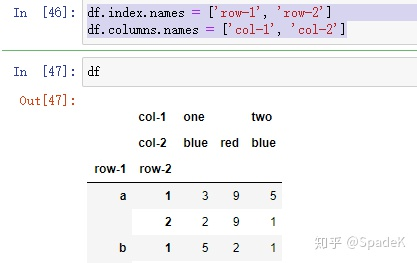交换索引df2 = df.swaplevel('row-1', 'row-2')#交换索引
df2
df.sum(level=0)#根据第一级索引求和
df.sum(level=1)#根据第二级索引求和
索引列的转换df = pd.DataFrame({
'a': range(7),
'b': range(7, 0, -1),
'c': ['one', 'one', 'one', 'two', 'two', 'two', 'two'],
'd': [0, 1, 2, 0, 1, 2, 3]
})
df
df.set_index('c')#把c这一列当作索引值
df2 = df.set_index(['c', 'd'])#把c,d都设置为索引值
df2
df3 = df2.reset_index().sort_index('columns')#转换回来换成一个平面的dataframne
df3
分组计算分组运算计算三步： 拆分->应用->合并拆分：根据什么分组（按照键值（key）或者分组变量将数据分组。）应用：每个分组进行什么样的运算（对于每组应用我们的函数，这一步非常灵活，可以是python自带函数，可以是我们自己编写的函数。）合并：把每个分组的运算合并起来（将函数计算后的结果聚合。）核心：1.不论分组键是数组、列表、字典、Series、函数，只要其与待分组变量的轴长度一致都可以传入groupby进行分组。2.默认axis=0按行分组，可指定axis=1对列分组。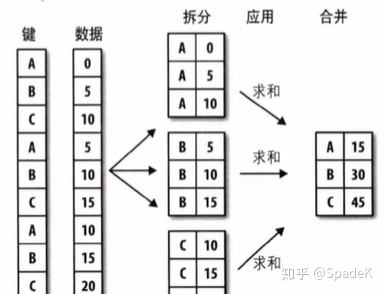1. 对Series进行分组import pandas as pd
import numpy as np
df = pd.DataFrame({'key1': ['a', 'a', 'b', 'b', 'a'],
'key2': ['one', 'two', 'one', 'two', 'one'],
'data1': np.random.randint(1, 10, 5),
'data2': np.random.randint(1, 10, 5)})
df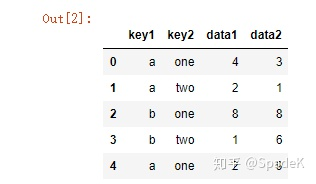grouped = df['data1'].groupby(df['key1'])#用key1进行分组
grouped
实际上，在这一步，我们并没有进行任何计算仅仅是创建用key1分组后创建了一个groupby对象，我们后面函数的任何操作都是基于这个对象的。grouped = df['data1'].groupby(df['key1']).mean()#用key1分组计算data1平均值
grouped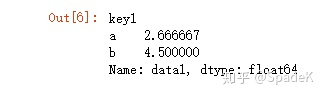语法很简单，但是这里需要注意grouped的数据类型，它不再是一个数据框，而是一个groupby对象df['data1'].groupby([df['key1'], df['key2']]).mean() #创建有多层索引的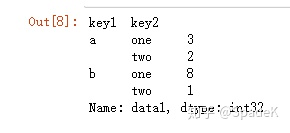2. 对DataFrame进行分组#对dtaframe进行分组
df.groupby('key1').mean()
means = df.groupby(['key1', 'key2']).mean()['data1']
means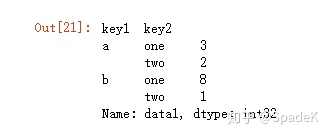通过unstack方法进行结果重塑：means.unstack()#生成的就是一个dataframe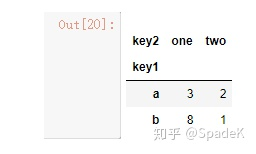对分组进行迭代GroupBy对象支持迭代操作，会产生一个由分组变量名和数据块组成的二元元组：for name, group in df.groupby('key1'):#支持python迭代器协议
#name分组的名字，group是是分组的数据
print (name)
print (group)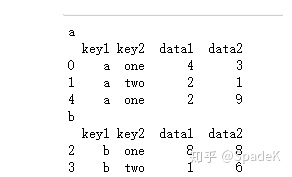list(df.groupby(['key1','key2']))#转化成list
3.通过字典进行分组#通过字典分组
df = pd.DataFrame(np.random.randint(1, 10, (5, 5)),
columns=['a', 'b', 'c', 'd', 'e'],
index=['zrx', 'dbw', 'lemon', 'mg', 'hzg'])

df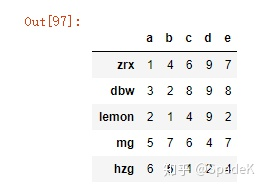df.loc[ 1:3] = np.NaN  #弄掉其中两行数据
df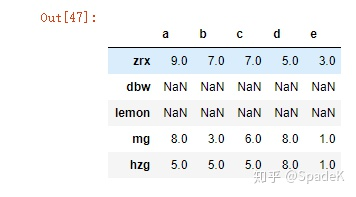想按列进行聚合我们根据实际情况，对列名建立字典，然后将此字典传入groupby，切记指定axis=1,因为我们是对列进行分组聚合：mapping = {'a': 'apple', 'b': 'banana', 'c': 'candy', 'd': 'apple', 'e': 'banana'}#对列名建立字典，列名进行分组
grouped = df.groupby(mapping, axis=1) #然后将此字典传入groupby
grouped.sum()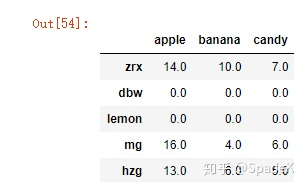grouped.size() #每个有几列
4.通过函数进行分组df = pd.DataFrame(np.random.randint(1, 10, (5, 5)),
columns=['a', 'b', 'c', 'd', 'e'],
index=['zrx', 'dbw', 'lemon', 'mg', 'hzg'])
def _dummy_group(idx):
print (idx)
return (idx)#返回索引的值
df.groupby(_dummy_group)#按行分组，所以每一行索引不一样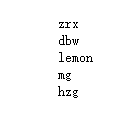def _dummy_group(idx):
print (idx)
return (len(idx))
df.groupby(_dummy_group).size()#分组的name的长度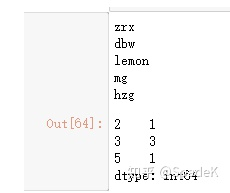5.根据索引级别分组刚刚我们的数据索引只有一级，当数据有多级索引时，可以通过level指定我们想要分组的索引，注意要使用axis=1表示按列：#多级索引进行分组
columns = pd.MultiIndex.from_arrays([['rx', 'rx', 'rx', 'bw', 'bw'],['t-shirt', 'skirt', 'dress', 'shoes', 'underwear']], names=['name', 'type'])
df = pd.DataFrame(np.random.randint(1, 10, (5,5)), columns=columns)
df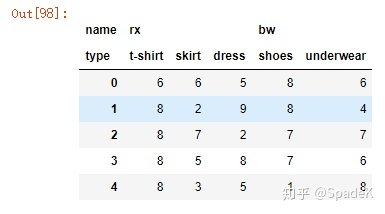我们名字进行分组求和：df.groupby(level='name', axis=1).sum()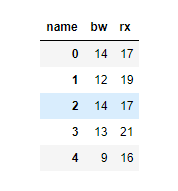ps：转自CSDN博主：zrx1236
展开全文对于以下数据帧：StationID  HoursAhead    BiasTempSS0279           0          10SS0279           1          20KEOPS            0          0KEOPS            1          5BB               0          5BB               1          5我想买一些像：StationID  BiasTempSS0279     15KEOPS      2.5BB         5我知道我可以写这样的脚本来得到想要的结果：def transform_DF(old_df,col):list_stations = list(set(old_df['StationID'].values.tolist()))header = list(old_df.columns.values)header.remove(col)header_new = headernew_df = pandas.DataFrame(columns = header_new)for i,station in enumerate(list_stations):general_results = old_df[(old_df['StationID'] == station)].describe()new_row = []for column in header_new:if column in ['StationID']:new_row.append(station)continuenew_row.append(general_results[column]['mean'])new_df.loc[i] = new_rowreturn new_df但我想知道熊猫身上是否有更直截了当的东西。
展开全文• 在将数据集准备好之后，通常的任务就是计算分组统计或生成透视表。pandas提供了一个灵活高效的gruopby功能，它使你能以一种自然的方式对数据集进行切片、切块、摘要等操作。关系型数据库和SQL(Structured Query ...
• 如果你想要利用python来学习数据分析和机器学习，你将很有可能使用非常强大的Pandas库。Pandas是python的一个可供数据操纵和分析的开源库。今天的内容是小编带你对Pandas进行简单的入门。1 读取数据这有一种很简单的...
• pandas提供了比较灵活的groupby分组接口，同时我们也可以使用pivot_table进行透视处理。1.分组 分组函数groupby，对某列数据进行分组，返回一个Groupby对象。分组在进行groupby分组后，我们可以对分组对象进行各种.....
• 前言上一篇（戳我查看）Pandas的语句介绍到DataFrame的滑片，这篇就继续介绍Pandas中的一些基本语句。数据清洗df.columns = ['a','b','c'] # 重命名列名称 pd.isnull() # 检查是否为null，为nul...
• I have te following pandas dataframe:data_df = pd.DataFrame({'ind':['la','p','la','la','p','g','g','la'],'dist':[10.,5.,7.,8.,7.,2.,5.,3.],'diff':[0.54,3.2,8.6,7.2,2.1,1.,3.5,4.5],'cas':[1.,2.,3.,4.,5...
• 回顾 在数据处理进阶pandas入门(十六)中，我们介绍了pandas中分组方法groupby()的各种分组方式以及多计算方法agg()的基本概念与用法。今天我们讲一下对groupby()方法分组数据处理的两个方法：数据分组转换transform...
• 数据分组分组统计 - groupby功能① 根据某些条件将数据拆分成组② 对每个组独立应用函数③ 将结果合并到一个数据结构中Dataframe在行(axis=0)或列(axis=1)上进行分组，将一个函数应用到各个分组并产生一个新，然后...
• 首先，根据Pandas时间戳创建一个列：df = df.dropna()df['date'] = df.apply(lambda x: pd.Timestamp('{year}-{month}-{day}'.format(year=int(x.Year),month=int(x.Month),day=int(x.Day))),axis=1)接下来，根据Lat...
• 回顾 在数据处理进阶pandas入门(十四)中，我们介绍了pandas中的去重与替换，需要掌握去重方法duplicates()方法和drop_duplicates()方法以及替换方法replace()的基本用法。今天我们讲一下pandas中分组的相关内容。...
• 数据聚合与分组运算——GroupBy技术(1)，有需要的朋友可以参考下。...计算分组摘要统计，如计数、平均值、标准差，或用户自定义函数。对DataFrame的列应用各种各样的函数。应用组内转换或其他运算，如规格...
• 回顾一下,我需要一些能让我得到每n个元素的比率列的平均值的东西,如果符号的数量不能被n整除,它会得到每n个的平均值,最后得到提醒的平均值(如果number_of_symbols只是这个数字的平均值). 例如,结果看起来像(假设n =...
• TUSHARE金融与技术学习兴趣小组翻译整理 | 一只小绿怪兽在处理数据的过程中，知道如何对数据集进行分组、聚合操作...分组、聚合操作一般会同时出现，用于计算分组数据的统计或实现其他功能。本文会介绍如何利用Pa...
• pandas中Series和DataFrame基本操作。设有DataFrame结果的数据a如下所示：a bcone4 1 1two6 2 0three6 1 6一、查看数据(查看对象的方法对于Series来说同样适用)1.查看DataFrame前xx行或后xx行a=DataFrame(data);a....
• 说明Pandas 中文教程修订中，欢迎加微信 sinbam 提供建议、纠错、催更。查看更新日志Pandas 窗口函数，为了处理数字数据，Pandas 提供几种窗口函数，如移动窗口函数(rolling())，扩展窗口函数(expanding())，指数...
• 在用 Excel 处理数据时，经常会遇到需要计算平均值的情况。学校里各科成绩出来了，要统计平均分；不同的领导打分占比不一样，要计算加权总得分；单位内部年终评比相互打分，要去掉一个最高分、一个最低分再计算平均...
• i）添加用于存放各索引分组平均值的列假设我们想要为一个DataFrame添加一个用于存放各索引分组平均值的列。一个办法是先聚合再合并：先聚合：再合并：虽然这样也行，但是不太灵活。你可以将该过程看作利用np.me...
• 我有122864行数据。我在HDF5文件中存储数据。...我想得到两次点击应用程序之间的平均持续时间。在1283 2015-04-01 08:07:44.1317681284 2015-04-01 08:08:02.7526111285 2015-04-01 08:08:02.793380...
• 上节介绍获取行、列子集的方法，运用了range函数和python的切片语法，在数据分析中，总是会进行统计计算，很多基本统计计算有现成的库可用，或内置在语言中。下面以data数据可以先问下面几个问题。1.数据中每年的...
• 重复的处理利用drop_duplicates()函数删除数据表中重复多余的记录, 比如删除重复多余的ID.import pandas as pddf = pd.DataFrame({"ID": ["A1000","A1001","A1002", "A1002"],"departmentId": [60001,600...
• 其还有mean()的方法 如果有必要，其实就是原理就是对哪个类型，进行以谁为分组，然后进行统计该数值得和或者是平均值都可以。 如果是对进行分组的类别进行计算大小的话，就是使用.size()的方法。 七、Categorical按...
• 天空澄碧，纤云不染本文转载来源于微信公众号：数据仓库与Python大数据(ID：dw_zzxx)前言在几乎所有的数据仓库与数据分析挖掘项目中都会遇到缺失数据的存在，数据缺失会导致...一般情况下，我们会使用变量的平均值...
• import pandas as pd movie= pd.read_csv('movie.csv') movie.head() Rank Title Genre Description Director ... Runtime (Minutes) Rating Votes Revenue (Millions) Metascore ...python 数据分析 开发工具
• 几乎任何一个数据集都含缺失数据。缺失数据有多种表示形式。...Pandas中的NaN来自NumPy库，在NumPy中，缺失有几个表达形式：NaN、NAN或者nan，但它们都是等同的。缺失和其它类型的数据不同，实际......